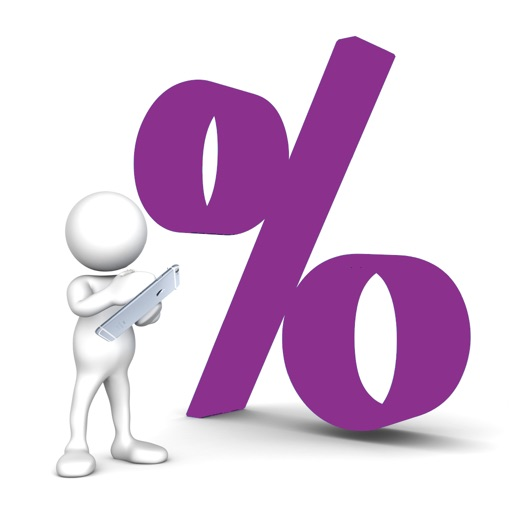All Calculator & UnitConverter# All Calculator & UnitConverter

Free
0

0 Ratings

Update Date

2020-04-19

Size

9.5 MB

### Screenshots for iPhone

iPhone
All Calculator & UnitConverter Description
All Calculator & UnitConverter application allows you to calculate:
1. Age Calculator
2. Date Calculator
2.1 Age Difference
2.2 Weekdays Between Two Dates
2.3 Leap Year
2.5 Subtract Days From Date
3. Fitness Calculator
3.1 BMR Calculator
3.2 FFMI Calculator
3.3 HWR Calculator
3.4 BP Calculator
3.5 Weight Watcher Calculator
3.6 BAC Calculator
3.7 Body Fat Calculator
3.8 Fat Percentage Calculator
3.9 Ideal Weight Calculator
4. Tip Calculator
5. Simple Interest Calculator
6. Compound Interest Calculator
7. GST Calculator
8. Tax Calculator
9. Percentage Calculator
10. Loan EMI Calculator
11. Unit Converter
11.1 Area Converter
11.2 Bits & Bytes Converter
11.3 Fuel Converter
11.4 Length Converter
11.5 Weight/Mass Converter
11.6 Speed Converter
11.7 Time Converter
11.8 Temperature Converter
11.9 Volume Converter
12. RD Maturity Calculator
13. STDR/FD Maturity Calculator
14. TDR/FD Maturity Calculator
15. TDR/FD Quarterly Interest Calculator
16. TDR/FD Monthly Interest Calculator
17. Simple Calculator

Save & Favourite:
√ Save Calculations
√ Update Saved Calculations
√ Make Favourite Calculations from Saved Calculations
√ Search Calculations from Saved and Favourite Calculations
√ Delete Calculations from Saved and Favourite Calculations

Charts:
√ Pie Chart
√ Bar Chart

Helps & Supports:
√ Provide help with example every where in app.

Other Features:
√ View calculation as a Pie chart and Bar chart with Save, Update, Favorite, Search and Delete Calculations
√ Easy to understand and calculate
√ Provide all at one place

All Calculator & UnitConverter:
=> Age Calculator:
Age Calculator allows you to calculate:
√ Age in Years, Months and Days
√ Next Birthday in Months and Days
√ Total Age Calculations
√ Upcoming 10 Birthdays
√ Total Years
√ Total Months
√ Total Weeks
√ Total Days
√ Total Hours
√ Total Minutes
√ Total Seconds
√ Calculate Your Age and Your Upcoming Birthdays with Time, Date, Year, Month, Week, Day, Hour, Minute & Second.

=> Date Calculator:
Date Calculator allows you to calculate:
√ Age Difference in Years, Months and Days
√ Weekdays Between Two Dates and Total Days
√ Leap Year Calculation with Days in Particular Month and Total Days in Year
√ Date + Days = New Date
√ Date - Days = New Date

=> Fitness Calculator:
Fitness Calculator allows:
√ You don't ever have to worry about the cooked food amounts you consume as a calorie tracker - since fitness calculator makes this step extra easy for you with one of our calculator.

=> Simple Interest Calculator
Simple Interest Calculator allows you to calculate:
√ Simple Interest for Years, Months and also for Days.

=> Compound Interest Calculator
Compound Interest Calculator allows you to calculate:
√ Compound Interest for Compounded:
√ Yearly
√ Semi-Annually
√ Quarterly
√ Monthly
√ Bi-Weekly
√ Weekly
√ Daily

=> Percentage Calculator
Percentage Calculator allows you to calculate:
√ Percentage
√ Shopping discount
√ Profit increase
√ Loss Decrease
√ What percent of amount is
√ What amount of percent is

=> Loan EMI Calculator
Loan EMI Calculator allows you to calculate:
√ Loan EMI
√ Monthly Instalment
√ Statistics
√ Result with Pie Chart and Bar Chart

=> Unit Converter
Unit Converter allows you to convert:
√ Area, Bits & Bytes, Fuel, Length, Weight/Mass, Speed, Time, Temperature, Volume etc...

=> FD and RD Calculator
Fixed Deposit (FD) and Recurring Deposit (RD) Interest Calculator allows you to calculate:
√ RD Maturity Calculator for Years and Months.
√ STDR/FD Maturity Calculator for Interest Frequency Yearly, Semi-Annually, Quarterly, Monthly, Bi-Weekly, Weekly and also for Daily
√ TDR/FD Maturity Calculator for Years, Months and also for Days.
√ TDR/FD Quarterly Calculator
√ TDR/FD Monthly Calculator

=> Simple Calculator
Simple Calculator allows you to calculate:
√ Simple Calculation with ×, ÷, +, - etc...
All Calculator & UnitConverter 1.0 Update
2020-04-19 Version History
More
Price:
Free
Version:
1.0
Size:
9.5 MB
Genre:
Productivity
Update Date:
2020-04-19
Developer:
girish chovatiya
Language:
English
More
You May Also Like
Developer Apps